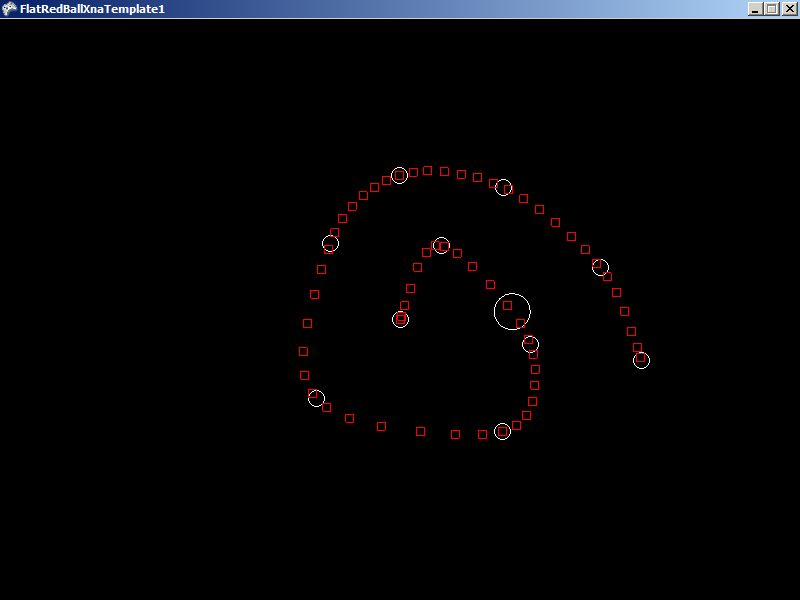# FlatRedBall.Math.Splines.Spline.GetPositionAtLengthAlongSpline

## Introduction

The GetPositionAtLengthAlongSpline method can be used to determine a position along the Spline after having traveled a certain distance.

## When is this method used?

Splines are used to define the path that an object moves along. Splines define both a curved path to follow as well as the velocities at each point. Therefore, when using the GetPositionAtTime method to move an object along a Spline, the object may speed up and slow down according to the velocity at a given spot along the Spline.

In some cases you may want to move along the Spline at a constant speed. To do this, you will want to use the path defined by the Spline, but not its velocity. If your object moves at a constant rate along a Spline, then the amount of time spent moving along the Spline is directly related to the length it has traveled along the Spline. The GetPositionAtLengthAlongSpline can be used to convert this length along a given Spline to a position.

## Code Example

The following example loads a SplineList, pulls the first Spline out of it, then gets the position along the Spline at a given length.

```using FlatRedBall.Math.Geometry;
using FlatRedBall.Math.Splines;
```

Add the following at class scope:

```Spline mSpline;
Circle mCircle;
```

Add the following to initialize after initializing FlatRedBall:

```SplineList splineList = FlatRedBallServices.Load<SplineList>(@"Content\ExampleSpline.splx");
```
```mSpline = splineList;
mSpline.CalculateAccelerations();
mSpline.Visible = true;
```
```// You must calculate distance/time relationships before calling GetPositionAtLengthAlongSpline
double totalTime = mSpline.Duration;
int numberOfSubdivisions = 200;
mSpline.CalculateDistanceTimeRelationships((float)(totalTime / numberOfSubdivisions));
```
```mCircle = ShapeManager.AddCircle();
```

```// First we determine how far along the spline we want to get the position at.
// CurrentTime gives us the number of seconds since the game started.
float desiredVelocity = 2; // increase to go faster
float distanceTraveled = (float)TimeManager.CurrentTime * desiredVelocity;
```
```// If using March 2010 or earlier, convert the length to time
double timeOnSpline = mSpline.GetTimeAtLengthAlongSpline(distanceTraveled);
// now convert that time to actual position:
mCircle.Position = mSpline.GetPositionAtTime(timeOnSpline);
```
```// Else if using April 2010 or newer, one function does it all
mCircle.Position = mSpline.GetPositionAtLengthAlongSpline(distanceTraveled);
```